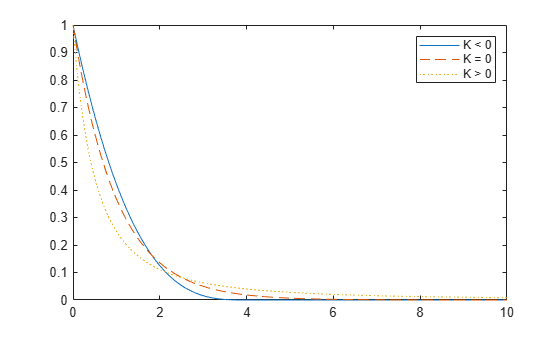Generalized Pareto Distribution

Definition

The probability density function for the generalized Pareto distribution with shape parameter k0, scale parameter σ, and threshold parameter θ, is

$y\text{​}\text{​}\text{\hspace{0.17em}}\text{\hspace{0.17em}}=\text{​}\text{\hspace{0.17em}}f\left(x|k,\sigma ,\theta \right)=\text{​}\text{​}\text{​}\text{​}\text{​}\text{​}\text{\hspace{0.17em}}\left(\frac{1}{\sigma }\right){\left(1+k\frac{\left(x-\theta \right)}{\sigma }\right)}^{-1-\frac{1}{k}}$

for θ < x, when k > 0, or for θ < x < θσ/k when k < 0.

For k = 0, the density is

$y\text{​}\text{​}\text{\hspace{0.17em}}\text{\hspace{0.17em}}=\text{​}\text{\hspace{0.17em}}f\left(x|0\text{​},\sigma ,\theta \right)=\text{​}\text{​}\text{​}\text{​}\text{​}\text{​}\text{\hspace{0.17em}}\left(\frac{1}{\sigma }\right){e}^{-\frac{\left(x-\theta \right)}{\sigma }}$

for θ < x.

If k = 0 and θ = 0, the generalized Pareto distribution is equivalent to the exponential distribution. If k > 0 and θ = σ/k, the generalized Pareto distribution is equivalent to the Pareto distribution with a scale parameter equal to σ/k and a shape parameter equal to 1/k.

Background

Like the exponential distribution, the generalized Pareto distribution is often used to model the tails of another distribution. For example, you might have washers from a manufacturing process. If random influences in the process lead to differences in the sizes of the washers, a standard probability distribution, such as the normal, could be used to model those sizes. However, while the normal distribution might be a good model near its mode, it might not be a good fit to real data in the tails and a more complex model might be needed to describe the full range of the data. On the other hand, only recording the sizes of washers larger (or smaller) than a certain threshold means you can fit a separate model to those tail data, which are known as exceedances. You can use the generalized Pareto distribution in this way, to provide a good fit to extremes of complicated data.

The generalized Pareto distribution allows a continuous range of possible shapes that includes both the exponential and Pareto distributions as special cases. You can use either of those distributions to model a particular dataset of exceedances. The generalized Pareto distribution allows you to “let the data decide” which distribution is appropriate.

The generalized Pareto distribution has three basic forms, each corresponding to a limiting distribution of exceedance data from a different class of underlying distributions.

• Distributions whose tails decrease exponentially, such as the normal, lead to a generalized Pareto shape parameter of zero.

• Distributions whose tails decrease as a polynomial, such as Student's t, lead to a positive shape parameter.

• Distributions whose tails are finite, such as the beta, lead to a negative shape parameter.

The generalized Pareto distribution is used in the tails of distribution fit objects of the paretotails object.

Parameters

If you generate a large number of random values from a Student's t distribution with 5 degrees of freedom, and then discard everything less than 2, you can fit a generalized Pareto distribution to those exceedances.

rng default  % For reproducibility
t = trnd(5,5000,1);
y = t(t > 2) - 2;
paramEsts = gpfit(y)
paramEsts = 1×2

0.1445    0.7225

Notice that the shape parameter estimate (the first element) is positive, which is what you would expect based on exceedances from a Student's t distribution.

hist(y+2,2.25:.5:11.75);
h = findobj(gca,'Type','patch');
h.FaceColor = [.8 .8 1];
xgrid = linspace(2,12,1000);
line(xgrid,.5*length(y)*...
gppdf(xgrid,paramEsts(1),paramEsts(2),2));Examples

Compute Generalized Pareto Distribution pdf

Compute the pdf of three generalized Pareto distributions. The first has shape parameter k = -0.25, the second has k = 0, and the third has k = 1.

x = linspace(0,10,1000);
y1 = gppdf(x,-.25,1,0);
y2 = gppdf(x,0,1,0);
y3 = gppdf(x,1,1,0);

Plot the three pdfs on the same figure.

figure;
plot(x,y1,'-', x,y2,'--', x,y3,':')
legend({'K < 0' 'K = 0' 'K > 0'});Embrechts, P., C. Klüppelberg, and T. Mikosch. Modelling Extremal Events for Insurance and Finance. New York: Springer, 1997.

 Kotz, S., and S. Nadarajah. Extreme Value Distributions: Theory and Applications. London: Imperial College Press, 2000.